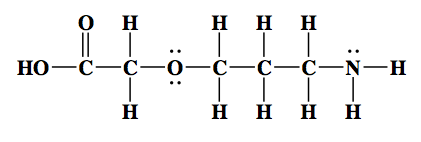Ch.20 - Organic ChemistryWorksheetSee all chapters
 Ch.1 - Intro to General Chemistry 2hrs & 53mins 0% complete Worksheet Ch.2 - Atoms & Elements 2hrs & 40mins 0% complete Worksheet Ch.3 - Chemical Reactions 3hrs & 25mins 0% complete Worksheet BONUS: Lab Techniques and Procedures 1hr & 38mins 0% complete Worksheet BONUS: Mathematical Operations and Functions 47mins 0% complete Worksheet Ch.4 - Chemical Quantities & Aqueous Reactions 3hrs & 30mins 0% complete Worksheet Ch.5 - Gases 3hrs & 47mins 0% complete Worksheet Ch.6 - Thermochemistry 2hrs & 28mins 0% complete Worksheet Ch.7 - Quantum Mechanics 2hrs & 35mins 0% complete Worksheet Ch.8 - Periodic Properties of the Elements 1hr & 57mins 0% complete Worksheet Ch.9 - Bonding & Molecular Structure 2hrs & 5mins 0% complete Worksheet Ch.10 - Molecular Shapes & Valence Bond Theory 1hr & 31mins 0% complete Worksheet Ch.11 - Liquids, Solids & Intermolecular Forces 3hrs & 40mins 0% complete Worksheet Ch.12 - Solutions 2hrs & 17mins 0% complete Worksheet Ch.13 - Chemical Kinetics 2hrs & 22mins 0% complete Worksheet Ch.14 - Chemical Equilibrium 2hrs & 26mins 0% complete Worksheet Ch.15 - Acid and Base Equilibrium 4hrs & 42mins 0% complete Worksheet Ch.16 - Aqueous Equilibrium 3hrs & 48mins 0% complete Worksheet Ch. 17 - Chemical Thermodynamics 1hr & 44mins 0% complete Worksheet Ch.18 - Electrochemistry 2hrs & 58mins 0% complete Worksheet Ch.19 - Nuclear Chemistry 1hr & 33mins 0% complete Worksheet Ch.20 - Organic Chemistry 3hrs 0% complete Worksheet Ch.22 - Chemistry of the Nonmetals 2hrs & 1min 0% complete Worksheet Ch.23 - Transition Metals and Coordination Compounds 1hr & 54mins 0% complete Worksheet

# Structural Formula

See all sections
Sections
Introduction to Organic Chemistry
Structural Formula
Chirality
Optical Isomers
Hydrocarbon
The Alkyl Group
Naming Alkanes
Naming Alkenes
Naming Alkynes
Alkane Reactions
Alkenes and Alkynes
Benzene Reactions
Functional Groups
Alcohol Reactions
Carboxylic Acid Derivative Reactions
Stereoisomers
Organic Chemistry Nomenclature
Benzene
Polymer

An organic compound can be written in different ways. Each one is a unique expression of the bonds each element is making.

###### Molecular Formulas

Concept #1: Structural Formula vs. Condensed Formula vs. Skeletal Formula.

Transcript

Hey guys! In this new video, we're going to take a look at structural formulas. We're going to say your typical organic molecule can be drawn in a few different ways. If we take for example pentane. Pentane’s formula is C5H12. There are different ways we can draw it. The three major ways that we can talk about it is our structural formula. In our structural formula, we show all the connections that each of the elements is making. We also call this our expanded formula. Expanded because we've blown it out where every single bond is shown. Remember, carbon is a tetravalent. Tetravalent, meaning that carbon wants to make four bonds when it's neutral.
Here if you take a look, each carbon in the center is making four bonds in some way either by making those bonds with hydrogens or with carbons around them. They're all making four bonds. Here, you can transition away from your structural formula to what's called your condensed formula. In your condensed formula, you basically just push the bonds back in around that central element. If you take a look, this carbon here, it is connected to one, two, three hydrogens so it's a CH3 group. There goes our CH3 group. This carbon here, it is connected to one, two carbons so it's a CH2 group. This carbon here is connected to one, two. It's also a CH2. This carbon here is connected to one, two. It is also a CH2. Then finally, the carbon at the end is connected to one, two, three hydrogens that's why it's a CH3. We go from the structural or expanded formula to the condensed formula when we push the bonds inward. Everything is compact basically.
Then next we move to the skeletal formula. When you guys get to Organic, you'll also hear it being called by different name. We can hear that it's called by a new name which is called a kekule or kekule structure. K-E-K-U-L-E with a little accent mark above the e. Kekule or kekule depending on which professor you get because they pronounce them differently. That's a skeletal formula. How do we read something like this? What you need to realize here that every end is a carbon. Every end and every corner is a carbon. There's a carbon here, here, here, here and here. At both ends there's a carbon and basically at every edge there's a carbon. The rule about skeletal formula is that you cannot show carbons. Carbons are invisible. They're there and we just can't draw them. Also, any hydrogens connected to them are also invisible. The carbons are invisible and the hydrogens connected to them are invisible.
If you have an OH or an NH or some other group, if you have a different element than carbon and it has Hs on it, then you'd have to show that different element and you can show the hydrogens connected to that different element. Again, when it comes to our skeletal formula carbons are invisible and the hydrogen is connected to them are also invisible. The only way I could show those hydrogens is if those hydrogens were connected to something different from carbon such as a halogen, such as sulfur or phosphorus or oxygen or nitrogen.
Remember, we said that carbon is tetravalent, meaning it needs to make four bonds to be satisfied. So every and every corner is a carbon, so what you need to realize here is that if this is a carbon, we see it making one bond with another carbon. That must mean it has three hydrogens on it that we don't see. Why does it need three hydrogens? Because again, carbon is tetravalent. It needs to make four bonds. Then here, this carbon we see it making one bond, two bonds. That means it has two hydrogens we don't see to help it get to four bonds total. Same thing with this carbon. We see it's connected to one, two carbons so it must have two hydrogens that we don't see in order to get to four. Here, this carbon is making one, two bonds so it needs two more hydrogens to get to four. Then finally the end carbon, we see it making one bond here so it must have three hydrogens that we don't see.
You'll also hear about your professors talking about the ball and stick way of drawing these. That's with like models and stuff like that. But when it comes to actually taking the exam and doing the work either online or in class, these are the three predominant formulas that we use for any organic molecule. We can draw it as a structural formula where we expand all the bonds out. We can draw it as a condensed formula where we push the bonds in or we can draw it as a skeletal formula, where we do not show any carbons or the hydrogens connected to them.
Now that we've seen this, I want you guys to attempt to do some of these example questions. We’ll come back, take a look at each one, one by one, and see what's the best way to look at it. Remember, every corner, every edge is a carbon. I hope you guys a little bit on this first one. Every end, every edge is a carbon. All those that I’ve marked are carbons. Remember, carbon is tetravalent. What does tetravalent mean? Use that to determine the number of hydrogens on each of those carbons.
Once you figure out the total number of elements, then you can get the molecular formula. Also remember, here why do I have to show this hydrogen now? Because it's connected to an element different from carbon. It's connected to an oxygen, so now we can show it. This oxygen up here is different from carbon. It has no hydrogens on it. We know that hydrogen is not going to be invisible on it because again, if it’s an element different from carbon, we can show the hydrogens connected to it. So guys, try to attempt to do this question on your own. We'll come back and take a look at this first one here.

In the structural formula all the individual bonds are shown. This representation is sometimes called the expanded chemical formula.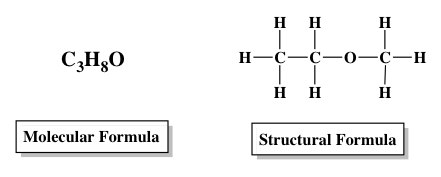In the condensed formula the bonds are pushed in to give a more compact representation of the bonds.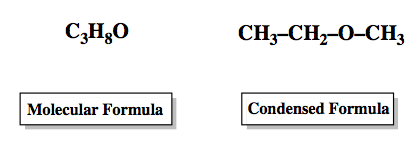In the skeletal formula all carbons and the hydrogens connected to them are not shown. Only elements different from carbon and the hydrogens connected to them can be represented.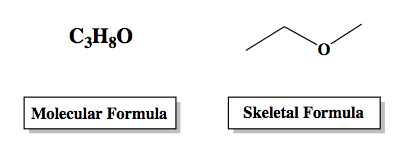Example #1: Determine the molecular formula for the following organic compound.

Transcript

Aright guys, so let's look at this example here. Every corner, every edge is a carbon. We know that carbon must make four bonds. This carbon here, we see it making one bond to that carbon so it must have three Hs on it to get to four. This carbon here, we see it making one, two, three, four bonds so it has no more room to accept any hydrogens, so it's fine. This is a carbon here. We see it making one bond so it must have three H's that we don't see to get it to four. Same with this carbon, we see it making one bond so it must have three hydrogens on it that we don't see. This carbon here is making two bonds, one, two, so it must have two hydrogens we don't see. Then this carbon here is making one, two, three, four bonds total so it's satisfied in terms of being tetravalent. It has no hydrogens on it at all.
Let's count how many total carbons we have. We have one, two, three, four, five, six carbons. so C6. Next, hydrogens. We have three, six, nine, eleven, twelve. And then how many oxygens do we have? We have two oxygens. This will be your molecular formula: C6H12O2. That would be the formula that you should have gotten for this first compound here. Now that we've done that one, try to do this one. This one isn't written as a skeletal formula. I've shown it as an expanded or structural formula, so this one should be a lot easier. Just literally count the number of elements and then give me the structural formula for. Come back. Let's take a look at it once you've done that.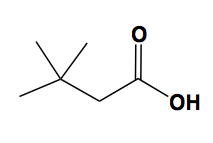Example #2: Convert the following structural formula into its condensed formula.Example #3: Determine the molecular formula for the following organic compound.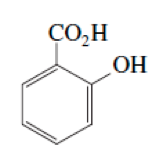Example #4: Determine the molecular formula for the following organic compound.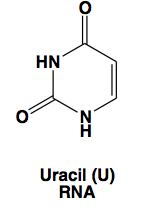Example #5: Convert the following structural formula into the carbon skeleton formula, otherwise known as a kekulé structure.